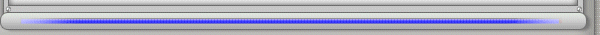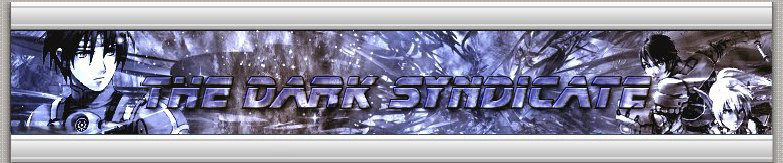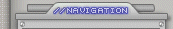» Information « Item Digits » Patches « Invisible Items Walk Through Doors God Equip Easy Level Max Money* Infinite Item Usage Fast Techniques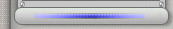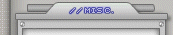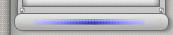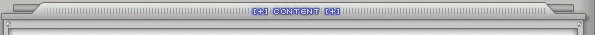******************************WEAPONS***************************** 00100 = Saber 000101 = Brand 000102 = Buster 000103 = Pallasch 000104 = Gladius 000105 = DB's SABER 000106 = KALADGOLG 000107 = DURANDAL 000200 = Sword 000201 = Gigush 000202 = Breaker 000203 = Claymore 000204 = Calibur 000205 = FLOWEN'S SWORD 000206 = LAST SURVIVOR 000207 = DRAGON SLAYER 000300 = Dagger 000301 = Knife 000302 = Blade 000303 = Edge 000304 = Ripper 000305 = BLADE DANCE 000306 = BLOODY ART 000307 = CROSS SCAR 000400 = Part =an 000401 = Halbert 000402 = Glaive 000403 = Berdys 000404 = Gungnir 000405 = BRIONAC 000406 = VJAYA 000407 = GAE BOLG 000500 = Slicer 000501 = Spinner 000502 = Cutter 000503 = Sawcer 000504 = D iska 000505 = SLICER OF ASSASSIN 000506 = DISKA OF LIBERATOR 000507 = DISKA OF BRAVEMEN 000600 = Handgun 000601 = Autogun 000602 = Lockgun 000603 = Railgun 000604 = Raygun 000605 = VARISTA 000606 = CUSTOM RAY Ver.00 000607 = BRAVACE 000700 = Rifle 000701 = Sniper 000702 = Blaster 000703 = Beam 000704 = Laser 000705 = V ISK'235W 000706 = WALS'MK2 000707 = JUSTY'23ST 000800 = Mechgun 000801 = Assault 000802 = Repeater 000803 = Gatling 000804 = Vulcan 000805 = M&A60 VISE 000806 = H&S25 JUSTICE 000807 = L&K15 COMBAT 000900 = Shot 000901 = Spread 000902 = Cannon 000903 = Launcher 000904 = Arms 000905 = CRUSH BULLET 000906 = METEOR SMASH 000907 = FINAL IMPACT 000A00 = Cane 000A01 = Stick 000A02 = Mace 000A03 = Club 000A04 = CLUB OF LACONIUM 000A05 = MACE OF ADAMAN 000A06 = CLUB OF ZUMIURAN 000B00 = Rod 000B01 = Pole 000B02 = Pillar 000B03 = Striker 000B04 = BATTLE VERGE 000B05 = BRAVE HAMMER 000B06 = ALIVE AQHU 000C00 = Wand 000C01 = Staff 000C02 = Baton 000C03 = Scepter 000C04 = FIRE SCEPTER:AGNI 000C05 = ICE STAFF:DAGON 000C06 = STORM VAND:INDRA 000D00 = PHOTON CLAW 000D01 = SILENCE CLAW 000D02 = NEI'S CLAW 000E00 = DOUBLE SABER 000E01 = STAG CUTLERY 000E02 = TWIN BRAND 000F00 = BRAVE KNUCKLE 000F01 = ANGRY FIST 000F02 = GOD HAND 000F03 = SONIC KNUCKLE 001000 = OROTIAGITO 001001 = AGITO (1975 Dousetsu) 001002 = AGITO (1983 Dousetsu) 001003 = AGITO (2001 Kikoku) 001004 = AGITO (1991 Kikoku) 001005 = AGITO (1977 Jou'un) 001006 = AGITO (1980 Tengai) 001100 = SOUL EATER 001101 = SOUL BANISH 001200 = SPREAD NEEDLE 001300 = HOLY RAY 001400 = INFERNO BAZOOKA 001500 = FLAME VISIT 001600 = AKIKO'S FRYING PAN 001700 = C-SORCERER'S CANE 001800 = S-BEAT'S BLADE 001900 = P-ARMS'S BLADE 001A00 = DELSABER'S BUSTER 001B00 = C-BRINGER'S RIFLE 001C00 = EGG BLASTER 001D00 = PSYCHO WAND 001E00 = HEAVEN PUNISHER 001F00 = LAVIS CANNON 002000 = VICTOR AXE 002100 = CHAIN SAWD 002200 = CADUCEUS 002300 = STING TIP 002400 = MAGICAL PIECE 002500 = TECHNICAL CROZIER 002600 = SUPPRESSED GUN 002700 = ANCIENT SABER 002800 = HARISEN BATTLE FAN 002900 = YAMIGARASU 002A00 = AKIKO'S WOK 002B00 = TOY HAMMER 002C00 = ELYSION 002D00 = RED SABER 002E00 = METEOR CUDGEL 002F00 = MONKEY KING BAR 002F01 = BLACK KING BAR 003000 = DOUBLE CANNON 003100 = HUGE BATTLE FAN 003200 = TSUMIKIRI J-SWORD 003300 = SEALED J-SWORD 003400 = RED SWORD 003500 = CRAZY TUNE 003600 = TWIN CHAKRAM 003700 = WOK OF AKIKO'S SHOP 003800 = LAVIS BLADE 003900 = RED DAGGER 003A00 = MADAM'S PARASOL 003B00 = MADAM'S UMBRELLA 003C00 = IMPERIAL PICK 003D00 = BERDYSH 003E00 = RED PARTISAN 003F00 = FLIGHT CUTTER 004000 = FLIGHT FAN 004100 = RED SLICER 004200 = HANDGUN:GULD 004300 = HANDGUN:MILLA 004400 = RED HANDGUN 004500 = FROZEN SHOOTER 004600 = ANTI ANDROID RIFLE 004700 = ROCKET PUNCH 004800 = SAMBA MARACAS 004900 = TWIN PSYCHOGUN 004A00 = DRILL LAUNCHER 004B00 = GULD MILLA 004C00 = RED MECHGUN 004D00 = BELRA CANNON 004E00 = PANZER FAUST 004F00 = SUMMIT MOON 005000 = WINDMILL 005100 = EVIL CURST 005200 = FLOWER CANE 005300 = HILDEBEAR'S CANE 005400 = HILDEBLUE'S CANE 005500 = RABBIT WAND 005600 = PLANTAIN LEAF 005601 = FATSIA 005700 = DEMONIC FORK 005800 = STRIKER OF CHAO 005900 = BROOM 005A00 = PROPHETS OF MOTAV 005B00 = THE SIGH OF A GOD 005C00 = TWINKLE STAR 005D00 = PLANTAIN FAN 005E00 = TWIN BLAZE 005F00 = MARINA'S BAG 006000 = DRAGON'S CLAW 006100 = PANTHER'S CLAW 006200 = S-RED'S BLADE 006300 = PLANTAIN HUGE FAN 006400 = CHAMELEON SCYTHE 006500 = YASMINKOV 3000R 006600 = ANO RIFLE 006700 = BARANZ LAUNCHER 006800 = BRANCH OF PAKUPAKU 006900 = HEART OF POUMN 006A00 = YASMINKOV 2000H 006B00 = YASMINKOV 7000V 006C00 = YASMINKOV 9200M 006D00 = MASER BEAM 006E00 = GAME MAGAZINE 006F00 = FLOWER BOUQUET 007000 = (S Rank Weapon) SABER 007100 = (S Rank Weapon) SWORD 007200 = (S Rank Weapon) BLADE 007300 = (S Rank Weapon) PARTISAN 007400 = (S Rank Weapon) SLICER 007500 = (S Rank Weapon) GUN 007600 = (S Rank Weapon) RIFLE 007700 = (S Rank Weapon) MECHGUN 007800 = (S Rank Weapon) SHOT 007900 = (S Rank Weapon) CANE 007A00 = (S Rank Weapon) ROD 007B00 = (S Rank Weapon) WAND 007C00 = (S Rank Weapon) TWIN 007D00 = (S Rank Weapon) CLAW 007E00 = (S Rank Weapon) BAZOOKA 007F00 = (S Rank Weapon) NEEDLE 008000 = (S Rank Weapon) SCYTHE 008100 = (S Rank Weapon) HAMMER 008200 = (S Rank Weapon) MOON 008300 = (S Rank Weapon) PSYCHOGUN 008400 = (S Rank Weapon) PUNCH 008500 = (S Rank Weapon) WINDMILL 008600 = (S Rank Weapon) HARISEN 008700 = (S Rank Weapon) J-BLADE 008800 = (S Rank Weapon) J-CUTTER 008900 = MUSASHI 008901 = YAMATO 008902 = ASUKA 008903 = SANGE & YASHA 008A00 = SANGE 008A01 = YASHA 008A02 = KAMUI 008B00 = PHOTON LAUNCHER 008B01 = GUILTY LIGHT 008B02 = RED SCORPIO 008C00 = TALIS 008C01 = MAHU 008C02 = HITOGATA 008C03 = DANCING HITOGATA 008C04 = .... 008D00 = NUG-2000 BAZOOKA 008E00 = S-BERILL'S HAND #0 008E01 = S-BERILL'S HAND #1 008F00 = FLOWEN'S SWORD 008F01 = 008F02 = 008F03 = 008F04 = 008F05 = 008F06 = 008F07 = 008F08 = 008F09 = 009000 = DB'S SWORD 009001 = 009002 = 009003 = 009004 = 009005 = 009006 = 009007 = 009008 = 009009 = 009100 = GIGUE BAZOOKA 009200 = GUARDIANNA 009300 = VIRIDIA CARD 009301 = GREENNILL CARD 009302 = SKYLY CARD 009303 = BRUEFULL CARD 009304 = PURPLENUM CARD 009305 = PINKAL CARD 009306 = REDRIA CARD 009307 = ORAN CARD 009308 = YELLOWBOZE CARD 009309 = WHITILL CARD 009400 = MORNING GLORY 009500 = PARTISAN OF LIGHTING 009600 = GAL WIND 009700 = ZANBA 009800 = RIKA'S CLAW 009900 = ANGLE HARP 009A00 = DEMOLITION COMET 009B00 = NEI'S CLAW 009C00 = RAINBOW BATON 009D00 = DARK FLOW 009E00 = DARK METEOR 009F00 = DARK BRIDGE 00A000 = G-ASSASIN'S SABERS 00A100 = RAPPY'S FAN 00A200 = BOOMA'S CLAW 00A201 = GOBOOMA'S CLAW 00A202 = GIGOBOOMA'S CLAW 00A300 = RUBY BULLET 00A400 = AMORE ROSE 00A500 = (S Rank Weapon) SWORD (Two Hands) 00A600 = (S Rank Weapon) LAUNCHER 00A700 = (S Rank Weapon) CARD 00A800 = (S Rank Weapon) KNUCKLE 00A900 = (S Rank Weapon) AXE 00AA00 = ??? 00AB00 = ??? 00AC00 = ??? ******************************ARMORS****************************** 010100 = Frame 010101 = Armor 010102 = Psy Armor 010103 = Giga Frame 010104 = Soul Frame 010105 = Cross Armor 010106 = Solid Frame 010107 = Brave Armor 010108 = Hyper Frame 010109 = Grand Armor 01010A = Shock Frame 01010B = King's Frame 01010C = Dragon Frame 01010D = Absorb Armor 01010E = Protect Frame 01010F = General Armor 010110 = Perfect Frame 010111 = Valiant Frame 010112 = Imperial Armor 010113 = Holiness Armor 010114 = Guardian Armor 010115 = Divinity Armor 010116 = Ultimate Frame 010117 = Celestial Armor 010118 = HUNTER FIELD 010119 = RANGER FIELD 01011A = FORCE FIELD 01011B = REVIVAL GARMENT 01011C = SPIRIT GARMENT 01011D = STINK FRAME 01011E = D-PARTS Ver1.01 01011F = D-PARTS Ver2.10 010120 = PARASITE WEAR:De Rol 010121 = PARASITE WEAR:Nelgal 010122 = PARASITE WEAR:Vajulla 010123 = SENSE PLATE 010124 = GRAVITON PLATE 010125 = ATTRIBUTE PLATE 010126 = FLOWEN'S FRAME 010127 = CUSTOM FRAME Ver.00 010128 = DB's ARMOR 010129 = GUARD WAVE 01012A = DF FIELD 01012B = LUMINOUS FIELD 01012C = CHU CHU FEVER 01012D = LOVE HEART 01012E = FLAME GARMENT 01012F = VIRUS ARMOR:Lafuteria 010130 = BRIGHTNESS CIRCLE 010131 = AURA FIELD 010132 = ELECTRO FRAME 010133 = SACRED CLOTH 010134 = SMOKING PLATE ******************************SHIELDS****************************** 010200 = Barrier 010201 = Shield 010202 = Core Shield 010203 = Giga Shield 010204 = Soul Barrier 010205 = Hard Shield 010206 = Brave Barrier 010207 = Solid Shield 010208 = Flame Barrier 010209 = Plasma Barrier 01020A = Freeze Barrier 01020B = Psychic Barrier 01020C = General Shield 01020D = Protect Barrier 01020E = Glorious Shield 01020F = Imperial Barrier 010210 = Guardian Shield 010211 = Divinity Barrier 010212 = Ultimate Shield 010213 = Spiritual Shield 010214 = Celestial Shield 010215 = INVISIBLE GUARD 010216 = SACRED GUARD 010217 = S-PARTS Ver1.16 010218 = S-PARTS Ver2.01 010219 = LIGHT RELIEF 01021A = SHIELD OF DELSABER 01021B = FORCE WALL 01021C = RANGER WALL 01021D = HUNTER WALL 01021E = ATTRIBUTE WALL 01021F = SECRET GEAR 010220 = COMBAT GEAR 010221 = PROTO REGENE GEAR 010222 = REGENERATE GEAR 010223 = REGENE GEAR ADV. 010224 = FLOWEN'S SHIELD 010225 = CUSTOM BARRIER Ver.00 010226 = DB'S SHIELD 010227 = RED RING 010228 = TRIPOLIC SHIELD 010229 = STANDSTILL SHIELD 01022A = SAFETY HEART 01022B = KASAMI BRACER 01022C = GODS SHIELD SUZAKU 01022D = GODS SHIELD GENBU 01022E = GODS SHIELD BYAKKO 01022F = GODS SHIELD SEIRYU 010230 = HUNTER'S SHELL 010231 = RICO'S GLASSES 010232 = RICO'S EARRING 010233 = BLUE RING 010234 = YELLOW RING 010235 = SECURE FEET 010236 = PURPLE RING 010237 = GREEN RING 010238 = BARRIER 010239 = BARRIER 01023A = RESTA MERGE 01023B = ANTI MERGE 01023C = SHIFTA MERGE 01023D = DEBAND MERGE 01023E = FOIE MERGE 01023F = GIFOIE MERGE 010240 = RAFOIE MERGE 010241 = RED MERGE 010242 = BARTA MERGE 010243 = GIBARTA MERGE 010244 = RABARTA MERGE 010245 = BLUE MERGE 010246 = ZONDE MERGE 010247 = GIZONDE MERGE 010248 = RAZONDE MERGE 010249 = YELLOW MERGE 01024A = RECOVERY BARRIER 01024B = ASSIST BARRIER 01024C = RED BARRIER 01024D = BLUE BARRIER 01024E = YELLOW BARRIER 01024F = WEAPONS GOLD SHIELD 010250 = BLACK GEAR 010251 = WORKS GUARD 010252 = RAGOL RING 010253 = BLUE RING 01025A = BLUE RING (NEW) 01025B = GREEN RING 010262 = GREEN RING (NEW) 010263 = YELLOW RING 01026A = YELLOW RING (NEW) 01026B = PURPLE RING 010272 = PURPLE RING (NEW) 010273 = WHITE RING 01027A = WHITE RING (NEW) 01027B = BLACK RING 010282 = BLACK RING (NEW) 010283 = WEAPONS SILVER SHIELD 010284 = WEAPONS COPPER SHIELD 010285 = ??? *******************************UNITS******************************* 010300 = Knight/Power 010301 = General/Power 010302 = Ogre/Power 010303 = God/Power 010304 = Priest/Mind 010305 = General/Mind 010306 = Angel/Mind 010307 = God/Mind 010308 = Marksman/Arm 010309 = General/Arm 01030A = Elf/Arm 01030B = God/Arm 01030C = Thief/Legs 01030D = General/Legs 01030E = Elf/Legs 01030F = God/Legs 010310 = Digger/HP 010311 = General/HP 010312 = Dragon/HP 010313 = God/HP 010314 = Magician/TP 010315 = General/TP 010316 = Angle/TP 010317 = God/TP 010318 = Warrior/Body 010319 = General/Body 01031A = Metal/Body 01031B = God/Body 01031C = Angel/Luck 01031D = God/Luck 01031E = Master/Ability 01031F = Hero/Ability 010320 = God/Ability 010321 = Resist/Fire 010322 = Resist/Flame 010323 = Resist/Burning 010324 = Resist/Cold 010325 = Resist/Freeze 010326 = Resist/Blizzard 010327 = Resist/Shock 010328 = Resist/Thunder 010329 = Resist/Storm 01032A = Resist/Light 01032B = Resist/Saint 01032C = Resist/Holy 01032D = Resist/Dark 01032E = Resist/Evil 01032F = Resist/Devil 010330 = All/Resist 010331 = Super/Resist 010332 = Perfect/Resist 010333 = HP/Restorate 010334 = HP/Generate 010335 = HP/Revival 010336 = TP/Restorate 010337 = TP/Generate 010338 = TP/Revival 010339 = PB/Amplifier 01033A = PB/Generate 01033B = PB/Create 01033C = Wizard/Technique 01033D = Devil/Technique 01033E = God/Technique 01033F = General/Battle 010340 = Devil/Battle 010341 = God/Battle 010342 = CURE POISON 010343 = CURE SHOCK 010344 = CURE PARALYSIS 010345 = CURE CONFUSION 010346 = CURE SLOW 010347 = CURE FROZEN 010348 = ??? 010349 = AI RAPPY   (UNIT = POWER + 150) 01034A = AI RAPPY   (UNIT = POWER + 150) 01034B = AI RAPPY   (UNIT = POWER + 150) ******************************MAGS****************************** 020000 = Mag 020100 = Varuna 020200 = Mitra 020300 = Surya 020400 = Vayu 020500 = Varaha 020600 = Kama 020700 = Ushasu 020800 = Apsaras 020900 = Kumara 020A00 = Kaitabha 020B00 = Tapas 020C00 = Bhirava 020D00 = Kalki 020E00 = Rudra 020F00 = Marutah 021000 = Yaksa 021100 = Sita 021200 = Garuda 021300 = Nandin 021400 = Ashvinau 021500 = Ribhava 021600 = Soma 021700 = Ila 021800 = Durga 021900 = Vritra 021A00 = Namuci 021B00 = Sumba 021C00 = Naga 021D00 = Pitri 021E00 = Kabanda 021F00 = Ravana 022000 = Marica 022100 = Soniti 022200 = Preta 022300 = Andhaka 022400 = Bana 022500 = Naraka 022600 = Madhu 022700 = Churel 022800 = RoboChao 022900 = Opa-Opa 022A00 = Pian 022B00 = Chao 022C00 = CHU CHU 022D00 = KAPU KAPU 022E00 = ANGEL'S WING 022F00 = DEVIL'S WING 023000 = ELENOR 023100 = MARK3 023200 = MASTER SYSTEM 023300 = GENESIS 023400 = SEGA SATURN 023500 = DREAMCAST 023600 = HAMBURGER 023700 = PANZER'S TAIL 023800 = DAVIL'S TAIL 023900 = 023A00 = RATI 023B00 = 023C00 = 023D00 = 023E00 = 023F00 = 024000 = 024100 = 024200 = 024300 = 024400 = ******************************ITEMS****************************** 030000 = Monomate 030001 = Dimate 030002 = Trimate 030100 = Monofluid 030101 = Difluid 030102 = Trifluid 030200 = Disk:Foie Lv.1 030300 = Sol Atomizer 030400 = Moon Atomizer 030500 = Star Atomizer 030600 = Antidote 030601 = Antiparalysis 030700 = Telepipe 030800 = Trap Vision 030900 = Scape Doll 030A00 = Monogrinder 030A01 = Digrinder 030A02 = Trigrinder 030B00 = Power Material 030B01 = Mind Material 030B02 = Evade Material 030B03 = HP Material 030B04 = TP Material 030B05 = Def Material 030B06 = Luck Material 030C00 = Cell Of MAG 502 030C01 = Cell Of MAG 213 030C02 = Parts Of RoboChao 030C03 = Heart Of Opa Opa 030C04 = Heart Of Pian 030C05 = Heart Of Chao 030D00 = Sorcerer's Right Arm 030D01 = S-beat's Arms 030D02 = P-arm's Arms 030D03 = Delsabre's Right Arm 030D04 = C-bringer's Right Arm 030D05 = Delsabre's Left Arm 030D06 = S-red's Arms 030D07 = Dragon's Claw 030D08 = Hildebear's Head 030D09 = Hildeblue's Head 030D0A = Parts of Baranz 030D0B = Belra's Right Arms 030D0C = GIGUE'S ARMS 030D0D = S-BERILL'S ARMS 030D0E = G-ASSASIN'S ARMS 030D0F = BOOMA'S RIGHT ARMS 030D10 = GOBOOMA'S RIGHT ARMS 030D11 = GIGOBOOMA'S RIGHT ARMS 030D12 = GAL WIND 030D13 = RAPPY'S WING 030D14 = ??? 030E00 = BERILL PHOTON 030E01 = PARASITIC GENE FLOW 030E02 = MAGICSTONE IRITISTA 030E03 = BLUE BLACK STONE 030E04 = SYNCESTA 030E05 = MAGIC WATER 030E06 = PARASITIC CELL TYPE D 030E07 = MAGIC ROCK HEART KEY 030E08 = MAGIC ROCK MOOLA 030E09 = STAR AMPLIFIER 030E0A = BOOK OF HITOGATA 030E0B = HEART OF CHU CHU 030E0C = PART OF EGG BLASTER 030E0D = HEART OF ANGLE 030E0E = HEART OF DEVIL 030E0F = KIT OF HAMBERGER 030E10 = PANTHER'S SPIRIT 030E11 = KIT OF MARK3 030E12 = KIT OF MASTER SYSTEM 030E13 = KIT OF GENESIS 030E14 = KIT OF SEGA SATURN 030E15 = KIT OF DREAMCAST 030E16 = AMP. RESTA 030E17 = AMP. ANTI 030E18 = AMP. SHIFTA 030E19 = AMP. DEBAND 030E1A = AMP. 030E1B = AMP. 030E1C = AMP. 030E1D = AMP. 030E1E = AMP. 030E1F = AMP. 030E20 = AMP. 030E21 = AMP. 030E22 = AMP. 030E23 = AMP. 030E24 = AMP. 030E25 = AMP. 030E26 = HEART OF KAPUKAPU 030E27 = PHOTON BOOSTER 030E28 = ??? 030F00 = ADD SLOT 031000 = PHOTON DROP 031001 = PHOTON SPHERE 031002 = PHOTON CRYSTAL 031100 = BOOK OF KATANA 1 031101 = BOOK OF KATANA 2 031102 = BOOK OF KATANA 3 031200 = WEAPONS BRONZE BADGE 031201 = WEAPONS SILVER BADGE 031202 = WEAPONS GOLD BADGE 031203 = WEAPONS CRYSTAL BADGE 031204 = WEAPONS STEEL BADGE 031205 = WEAPONS ALUMINUM BADGE 031206 = WEAPONS LEATHER BADGE 031207 = WEAPONS BONE BADGE 031208 = LETTER OF APPRECATION 031209 = AUTOGRAPH ALBUM 03120A = VALENTINE'S CHOCOLATE 03120B = NEWYEAR'S CARD 03120C = CRISMAS CARD 03120D = BIRTHDAY CARD 03120E = PROOF OF SONIC TEAM 03120F = SPECIAL EVENT TICKET 031300 = PRESENT 031400 = CHOCOLATE 031401 = CANDY 031402 = CAKE 031403 = SILVER BADGE 031404 = GOLD BADGE 031405 = CRYSTAL BADGE 031406 = IRON BADGE 031407 = ALUMINUM BADGE 031408 = LEATHER BADGE 031409 = BONE BADGE 03140A = BONQUET 03140B = DECOCTION 031500 = CRISMAS PRESENT 031501 = EASTER EGG 031502 = JACK-O'S-LANTERN 031600 = DISK VOL.1 031601 = DISK VOL.2 031602 = DISK VOL.3 031603 = DISK VOL.4 031604 = DISK VOL.5 031605 = DISK VOL.6 031606 = DISK VOL.7 031700 = HUNTERS REPORT 031701 = HUNTERS REPORT RANK A 031702 = HUNTERS REPORT RANK B 031703 = HUNTERS REPORT RANK C 031704 = HUNTERS REPORT RANK F     UPDATES: The site is under new management   [ Donations have reached \$16! ] [ We would like to personally thank all of you who have donated.] Phantasy Star Online is legal copyrighted property of Sega Sammy Holdings. No data directly from the disc should EVER be uploaded to any website. (xbe, so on and so fourth)